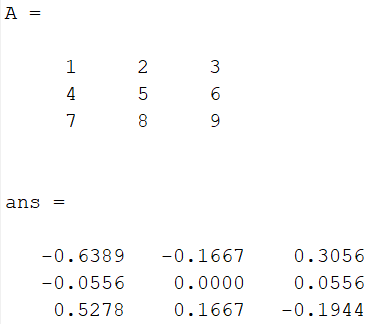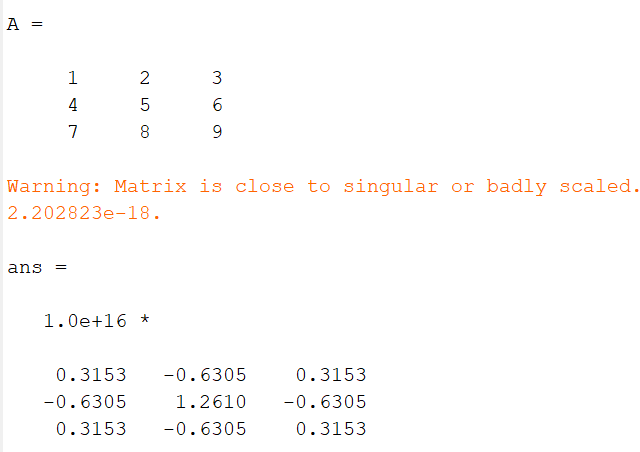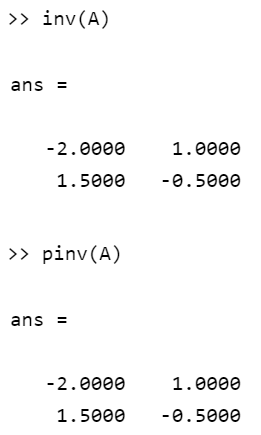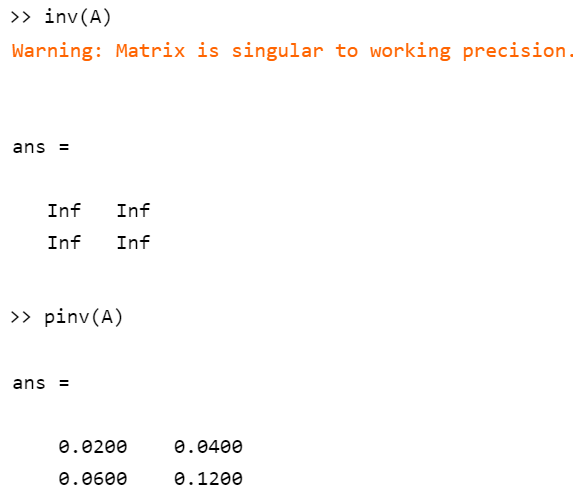# Difference between inv() and pinv() functions in MATLAB

• Last Updated : 21 Aug, 2021

While Handling data, used for Machine Learning, it’s become easy to do operations on data if stored in form of a Matrix. Where inverse is one of the important operations which is used in many places while handling data. Here are below two methods to find the inverse of a matrix used in different situations.

### pinv()

• It is used to handle Singular as well as Non-Singular Matrices, it refers to the pseudo-inverse of a matrix.
• The pinv() function involves the use of floating-point arithmetic.

Syntax:

pinv(A)

Where A is a Matrix of Order M x N.

Example:

## Matlab

 `% Matrix``A = [1 2 3; 4 5 6; 7 8 9]` `% Using inv()``pinv(A)`

Output:### inv()

• It is used to handle Non-Singular Matrices, it refers to inverse of a matrix.
• The inv() function doesn’t involve use of floating-point arithmetic.

Syntax:

inv(A)

Where A is a Matrix of Order M x N.

Example:

## Matlab

 `% Matrix``A = [1 2 3; 4 5 6; 7 8 9]` `% Using inv()``inv(A)`

Output:Let us Suppose there is a Matrix called A with some values in it is taken, we need to find the inverse of Matrix A using inbuilt Function, so both pinv(A) and inv(A) can be used to find the inverse of the Matrix. Consider the below program:

## Matlab

 `% Matrix``A = [1 2; 3 4]` `% Using inv()``inv(A)` `% Using pinv()``pinv(A)`

Output:As we can see both functions produces the same results for a normal matrix. Now we will use a singular matrix and apply both the functions to find its inverse.

## Matlab

 `% Matrix``A = [1 3; 2 6]` `% Using inv()``inv(A)` `% Using pinv()``pinv(A)`

Output:So, we can’t use inv() in case if Matrix is singular. But using the same Matrix, the inverse can be calculated using the pinv() function.

Both pinv() and inv() are used to find the inverse of matrices in MATLAB. However, the difference is that pinv refers to pseudo inverse and inv refers to inverse. Below are some key differences between both the functions:

## Table of Difference Between pinv() and inv()

My Personal Notes arrow_drop_up